﻿ Analysis of Some Properties of the Rasch Model in Physics EducationPublications are Open
Access in this journal
Article Versions
Export Article
• Normal Style
• MLA Style
• APA Style
• Chicago Style
Research Article
Open Access Peer-reviewed

### Analysis of Some Properties of the Rasch Model in Physics Education

Rubén Sánchez Sánchez , César Mora
American Journal of Educational Research. 2021, 9(3), 125-132. DOI: 10.12691/education-9-3-6
Received February 10, 2021; Revised March 16, 2021; Accepted March 25, 2021

### Abstract

In the educational field, there are several ways to teach and evaluate students, especially in knowledge of electrical circuits. In this work, we discuss some properties of the dichotomous Rasch model applied to the area of Physics Education, to evaluate the learning performance of high school students in knowledge of electrical circuits. The teaching exercise and data collection were carried out in a Mexican High School. Here we show how the Rasch model helps us to appreciate progress that students have in their knowledge about electrical circuits, when using an active teaching methodology in the classroom.

### 1. Introduction

There are several Rasch models, which can be of type dichotomous or polytomous, for example, a broader treatise that can be reviewed in the work written by Jürgen Rost 1 called The Growing Family of Rasch models.

There are also several applications of the model in some fields of knowledge and research 2, 3, 4, but here we want to focus on the field of education.

Rasch's model was derived between 1952 and 1961. Its history is described in detail by Andersen and Olsen 5. Georg Rasch designed his model, partly because of his research in finding the psychological profiles of recruit candidates from the Military Psychology Service (MPT) where Lieutenant Colonel F. Agersten served as its leader.

He made good progress in his model with the communication he had with Professor Ragnar Frisch in 1959, and finally managed to publish his ideas in the 1960 and 1961 years.

Here we want to discuss briefly a very simple Rasch model, which we can apply in the educational area for the purpose of evaluating the effectiveness achieved by using an active teaching methodology in Physics.

### 2. Dichotomous Rasch Model Applied in Education

To summarize a little bit, the mathematical equation behind a Rasch model simplified, that can be used in the educational field, it acquires the following mathematical expression: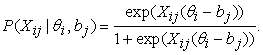(1)

The parameter Xij is known as the discrimination parameter and represents the slope of the curve drawn with equation (1), taking θi as independent variable, P as dependent variable, when θi and bj are equal then P becomes 1/2.

Let us assume for our case, an equal discrimination parameter to unity, then equation (1), simplifies to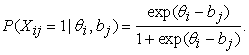(2)

The Rasch model (1) and (2) is called dichotomous because of the data type that can be handled when applying it in education. If in a test it is considered that the answer given by each student of a group, it can be right or wrong, then we are handling a data type that acquires two values: “1” (if the answer given by the student is correct) or “0” (if the answer given by the student is incorrect).

### 3. Description of Rasch’s Curves

In the Rasch model, various data collected are handled from the answers given by the students to a test of knowledge.

At a given hypothetical moment, we assume the case of a student answering a particular question in a test. We can say at that time, that the student has the possibility to answer correctly to the question depending on your level of knowledge on the subject that the question in turn deals with. The probability will also depend on the degree of difficulty that have the question. In this way, we have a double and independent vision, what is the probability of successfully answering the question of the test. On the one hand, this will depend on a parameter of difficulty, and on the other of an independent parameter of ability of the student, who answers the question.

Rasch found the most convenient way to express this fact mathematically, after studying the properties of mathematical independence that have certain parameters within the multiplicative model of Poisson 6.

Assuming the multiplicative independence of the parameter ability and difficulty parameter, is how can arrive at the mathematical expressions of the model dichotomous of equations (2) and (3) (If we also assume a discrimination parameter equal to unity).

Multiplicative independence can be expressed, using a mathematical property of exponents, so (2) can be to rewrite as follows.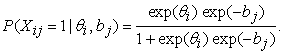(3)

Here we assume that there are i = 1, ..., N students with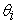ability parameters. And we assume j = 1, ..., M questions with parameters of difficulty bj.

The probability that student ith will have the correct answer to question or item jth is P(Xij = 1 |, bj).

Given a fixed difficulty of the question or item bj, we can calculate the probability curve, as a function of thestudent's ability parameter.

### 4. Brief Description of the Case of Study and of the Students

As we have said, The Rasch model is a very useful mathematical tool for the statistical analysis of the data in the educational field of research. To exemplify the use of the Rasch model in education, we are going to use the data of a particular case study as an example.

At the Xoclan campus, in Mérida Yucatán (Mexico), a class of electrical circuits was held in the first semester of 2020, using the didactic methodology known as Active Learning of Physics, with the PODS cycle that consist of the phases Predict-Observe-Discuss-Synthesize. The students are between 15 and 18 years old and are at the high school level, so the type of skills that are expected to have among these types of students are a degree of adequate oral and written comprehension, elementary skills of mathematics, arithmetic, and geometry. Regarding the skills in the field of physics, the students have taken courses in classical mechanics, where the elementary mathematics of addition subtraction, multiplication, division, and elementary algebraic operations are used (also called pre-calculus). They also have notions of handling elementary mechanical instruments. Also, they have high values of good social coexistence, cooperative work, and good communication between them.

In this study, didactic prototypes of electrical circuits were used, besides six questions from Sokoloff's Electric Circuits Conceptual Evaluation test (ECCE) 8 and, a sample of fifteen engineering students.

The way to proceed to collect data is as follows: First, the test is applied to the students to know your prior knowledge of electrical circuits.

Then the didactic methodology of Active Learning of Physics is applied to the group of students.

Once the students have learned the topic with this methodology, a later date is planned to return to apply the same test of electrical circuits.

The data are in dichotomous form, in tables of two values (0 and 1), the columns of each table represent the test questions, and the rows represents the students who brought the didactic methodology.

A “0” or a “1” is placed in each entry in the table, according to whether the respective student answered right to the indicated question.

Subsequently, the data analysis is performed, to get an idea of the effectiveness of the teaching method in this case.

We use the Rasch model for the evaluation of the student knowledge, and a programming language called R 7, which was designed by Robert Gentleman and Ross Ihaka, in Auckland, New Zealand. And we will make use of an R library called ltm, from the “latent trait model”.

The easiness parameters of the chosen test are calculated from six questions of the ECCE test for the pretest, and then for the posttest.

The easiness parameters would be the counterpart of the difficulty parameters, instead of measuring the level of difficulty of the item, these will measure the degree of easiness of each of the items of the test.

### 5. Didactic Prototypes

As we have said before, the students will develop several didactic prototypes based on the design of elementary electrical circuits in series and parallel. This for the students to identify the most outstanding parameters of each part of the circuits such as voltage, current and electrical resistance.

• Figure 1. Electric circuit prototype, with a measurement instrument (M), a power source (S) of 120 volts of altern current, and 5 bulbs: B1, B2, B3, B4, B5, connected in series.

The Figure 1 shows the suggested didactic prototype to analyze the parameters of a series circuit, in this case the total current of the circuit.

• Figure 2. Electric circuit prototype, with a measurement instrument (M), a power source (S) of 120 volts of altern current, and 5 bulbs: B1, B2, B3, B4, B5, connected in parallel

In Figure 2, we suggest a prototype of a parallel circuit with light bulbs, and a connected measuring instrument.

In Figure 3, we show a prototype for measuring the average voltage of one of the bulbs of a parallel circuit.

• Figure 3. Electric circuit prototype, with a measuring instrument (M), a power source (S) of 120 volts of altern current, and 5 bulbs: B1, B2, B3, B4, B5, connected in parallel

In Figure 4, we show another prototype of an electrical circuit with series resistors, and a connected measuring instrument.

• Figure 4. Electric circuit prototype, with a measurement instrument (M), a power source (S) of 120 volts of direct current, and 5 resistances: R1, R2, R3, R4, R5, connected in series.
• Figure 5. Electric circuit prototype, with a measuring instrument (M), a power source (S) of 120 volts of direct current, and 5 resistances: R1, R2, R3, R4, R5, connected in parallel

Finally, in Figure 5, we show another example of a didactic prototype for resistors connected in parallel and a measuring instrument connected to record the voltage of one of the resistors.

### 6. Recollection of Data

We can collect the data in excel sheets, as in Figure 6 and Figure 7. The Figure 6 shows the result of the questions answered, from the test, for the pretest; and Figure 7 are the responses which corresponds to the posttest phase.

• Figure 6. Recollected data for a sample group of 15 students, in a pretest phase

Then the data is entered in R, with the readxl library. Later the easiness parameters are calculated for the pretest and posttest of the same test and the same students’ group, using the latent trait model.

Here you can already make an assessment or take an opinion about the effectiveness of the didactic methodology for this case study and considering the values obtained from the easiness parameters of the test questions, both in the pretest phase and in the posttest, for the same sample of students.

• Figure 7. Recollected data for a sample group of 15 students, in a posttest phase

We can get the software R from the website: www.r-project.org 9 that can help us with the statistical computing and the plotting of graphs involved in the Rasch model. In the next section we will describe how our collected data can be entered within an R session, for further processing, using the Rasch model.

### 7. Data input for R

We have collected the data independently in Excel worksheets, to enter our data from the Excel sheets into an internal format that R can understand, we will use the readxl library. This library will help us in the management of the data, allowing its adequate capture in a suitable format, which R can understand. For this, first we install the library in R 10, 11 with the command.

The program R asks us from which place the library is going to be downloaded. After choosing a nearby repository, R proceed to download this library and let us know the place where it is installed on our computer.

After the library was installed in our system, we will proceed to invoke it within a session in R, with the following command.

Then R's current working directory is asked for, with the following command.

> getwd()

If the working directory is denoted as “~” (this symbol denotes the home directory of the user on a unix-like operating system) then we copy the Excel files of the data in this same directory.

Then the data from a table is entered into an internal R format, with the following order.

The data in the tables is stored in the variables data.pretest and data.posttest in a format that R calls a tibble, for later use.

### 8. Calculation of the Easiness Parameters of the Selected Test

The calculation of the easiness parameters is achieved with the library ltm 12, 13. First ltm is installed in a similar way to readxl, with the following order.

> install.packages("ltm")

and then this library is loaded in the current session with the command.

> library(ltm)

If we want to find the easiness parameters for the phase of the pretest, of our group and the selected test and save the results in the variable rasch.pretest. the function rasch() is used from the ltm library, as follows

> rasch.pretest

<- rasch(datos.pretest, cbind(ncol(datos.pretest)+1, 1))

The variable, rasch.pretest will contain the easiness parameters of the test applied, in the pretest phase, for our sample from students.

The Table 1 resumes the results of the easiness parameters for the pretest phase of our sample group, with the select questions of ECCE test.

We repeat the same procedure for the posttest data, and we obtain the easiness parameters of the same test and for the same sample of students, but in the posttest phase, once we have applied the didactic methodology to students, to teach them the elementary concepts of electrical circuits.

> rasch.posttest

<- rasch(datos.posttest, cbind(ncol(datos.posttest)+1, 1))

We observe that the level of easiness of each test question has changed, and in general it has incremented, by an amount that can be appreciated. Which suggests to us that Active Learning of Physics has been very useful for students to learn elementary concepts of the series and parallel electrical circuits, which they learn, following simple didactic prototypes. The Table 2, resumes the results of the easiness parameters for the posttest phase of our sample group, with the select questions of ECCE test. In the Table 1 and Table 2, we have followed the criteria suggested by Baker and Kim 12, in the chapter 1 of their book.

### 9. Getting the Plots of the Rasch Model

The graphs of the Rasch model will be called Item Characteristic Curves (ICC), which are obtained from a model like (2), knowing the values of the easiness parameters. The equation 14 to compute the probability of successfully answering the question, is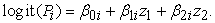(4)

Where Pi is the probability of a positive response in the ith item,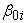are the easiness parameters,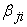(j=1, 2) are the discrimination parameters, and z1 and z2 are the latent variables. The later information can be accessed through the standard mechanism to get help in R for a package. In this case we can get the help of the ltm package online issuing the following command:

> help(ltm)

The R software can plot these curves for our test of selected questions and our sample of students, both in their pretest phase (showing graphically the level of knowledge of students, before learning), as in the posttest phase (which indicates the level of knowledge reached by the students, by applying the Active Learning of Physics to teach elementary electrical circuits).

The graph in Figure 8 shows the ICC for each question or item, in the pretest phase using a selected test, and with our sample of students.

The graph in Figure 9 shows the ICC for each question or item, in the posttest phase, using the selected test, and with the same sample of students.

• Figure 8. ICC curves of the sample group in the pretest phase
• Figure 9. ICC curves of the sample group in the posttest phase

If we compare the ICC curves of the posttest with those of the pretest, we find a progress or advancement in the level of probability to answer to the questions correctly for the posttest phase.

However, the numerical record, of the progress of the students, is saved in the parameter lists of easiness, which were obtained with the rasch() function of the ltm library, and that were later used to make the graphical representation in shape of the ICC curves of the same test items, for the phases of the pretest (Figure 8), and for the posttest (Figure 9).

The results are visually encouraging, as there is progress, which can be considered significant between the ICC curves of the pretest and the ICC of the posttest.

We can suggest that the Active Learning of Physics was a very good didactic teaching methodology for this case study.

### 10. The information Function

The way to estimate the reliability of our calculations is behind the information curves, associated with the characteristic curves of the item, the method was provided by Fisher 12, and there is a way to graph these curves using the same ltm library. Figure 10 shows the item information curves (IIC) for the pretest phase, and Figure 11 corresponds to the same type of curves for the posttest, and the same sample of students.

• Figure 10. IIC curves of the sample group in the pretest phase
• Figure 11. IIC curves of the sample group for the posttest phase

The mathematical relationship that links this information with the variance produced by the mathematical errors in the method is given by the following relation.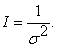(5)

Also, we have that the relationship with the standard error is connected by the equation.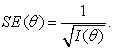(6)

And as we can see, the standard error SE depends on the value of the ability parameter that the student has, for this reason it is better to present this information in a graph.

### 11. Ability Parameters

We can calculate the ability parameters of the students from the data collected in the pretest of Figure 1. For this we use another R library called extended Rasch model (eRm) and the functions RM() and person.parameter(). First we use RM on the data in Figure 1, and then we apply the function person.parameter() to the return “object” of the first call. The steps in R, are as follows.

> library(eRm)

> pretest.results <-RM(datos.pretest)

> abilities<-person.parameter(pretest.results)

> summary(abilities)

We enter the calculated results of the ability parameters in Table 3, to complement our present study, for our sample of students.

The standard error and the data corresponding to 2.5% and 97.5% are also noted, with number of iterations 6 and 3 parameters and probability of collapse log -8.7966.

As we can see in the table, the students come initially with several of their ability parameters with a negative value.

### 12. Conclusions

We have seen, how the Rasch mathematical model, is useful to perform statistical analysis of the results in the test applied to the sample group of students. For this, it is necessary to know the model is its simple and dichotomous version, having the activities of the Active Learning of Physics for the study topic, apply the test, take the records of results before and after teaching (pretest and posttest, respectively).

And then use free software tools (like R and its libraries readxl, and ltm), to get results in the form of the easiness of the questions, both in the pretest and posttest phases for the same test.

Also, as we can see the level of the curves in Figure 8 and Figure 9, it has changed. Figure 8 shows the curves that measure the probability that students respond well to the corresponding test question. The level of the curves in the posttest is generally higher. Therefore, the analysis suggests an improvement in the level of knowledge of the students, once they have taken their electrical circuits classes using the didactic methodology of Active Physics Learning.

Table 1 and Table 2 also show a trend in the ability parameters to improve in the posttest, perhaps item 4 is not very favorable, as it remains with a very hard level of difficulty.

As for the initial abilities of the students, it can be said that they are initially below average, because there are several negative ability parameters.

If we gather all these individual observations, we are inclined to think that the didactic methodology of Active Physics Learning has given satisfactory results in the learning level of this sample of students.

An advantage that we can see that the analysis of the Rasch model has on Hake's gain 15, would be that Rasch model can give the information about the personalized performance of the students. While Hake's gain can be considered as a global average of the group or sample of students that we have.

### Acknowledgements

The authors wish to acknowledge the support received by the Consejo Nacional de Ciencia y Tecnología (CONACYT) of Mexico. Also wants to thank for the support received from the Instituto Politécnico Nacional, by COFAA grants and the research projects SIP20211283 and SIP20211347.

### References

Published with license by Science and Education Publishing, Copyright © 2021 Rubén Sánchez Sánchez and César MoraThis work is licensed under a Creative Commons Attribution 4.0 International License. To view a copy of this license, visit http://creativecommons.org/licenses/by/4.0/